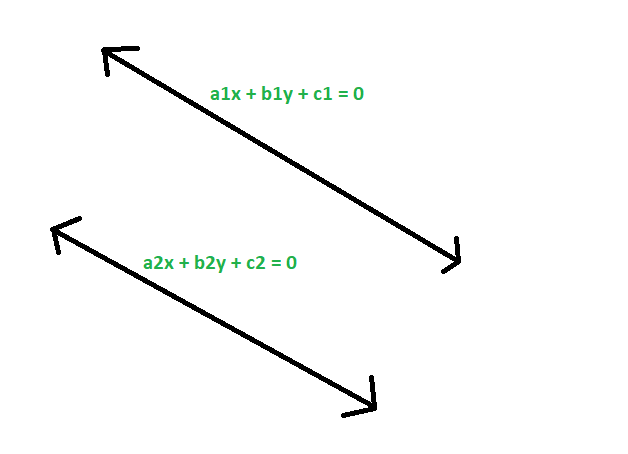# Check if given two straight lines are identical or not

Given two straight lines with co-efficients of there equations a1x + b1y + c1 = 0 and a2x + b2y + c2 = 0 respectively, the task is to check if the straight lines are identical or not.

Examples:

Input: a1 = -2, b1 = 4, c1 = 3, a2 = -6, b2 = 12, c2 = 9
Output: The given straight lines are identical

Input: a1 = 12, b1 = 3, c1 = 8, a2 = 7, b2 = -12, c2 = 0
Output: The given straight lines are not identicalApproach:

1. Given equations,
a1x + b1y + c1 = 0
a2x + b2y + c2 = 0
2. converting them to slope intercept form, we get
y = (-a1/b1)x +(-c1/b1)
y = (-a2/b2)x +(-c2/b2)
3. now, if the lines are identical then there slope and intercepts must be equal,
so,
-a1/b1 = -a2/b2
or, a1/a2 = b1/b2

also,
-c1/b1 = -c2/b2
so, c1/c2 = b1/b2
4. So, if two given straight lines are identical then there co-efficients should be proportional.Below is the implementation of the above approach

## C++

 // C++ program to check if  // given two straight lines  // are identical or not     #include  using namespace std;     // Function to check if they are identical  void idstrt(double a1, double b1,              double c1, double a2,              double b2, double c2)  {      if ((a1 / a2 == b1 / b2)          && (a1 / a2 == c1 / c2)          && (b1 / b2 == c1 / c2))          cout << "The given straight"              << " lines are identical"              << endl;         else         cout << "The given straight"              << " lines are not identical"              << endl;  }     // Driver Code  int main()  {      double a1 = -2, b1 = 4,             c1 = 3, a2 = -6,             b2 = 12, c2 = 9;      idstrt(a1, b1, c1, a2, b2, c2);      return 0;  }

## Java

 // Java program to check if  // given two straight lines  // are identical or not  class GFG  {     // Function to check if they are identical  static void idstrt(double a1, double b1,                      double c1, double a2,                      double b2, double c2)  {      if ((a1 / a2 == b1 / b2)          && (a1 / a2 == c1 / c2)          && (b1 / b2 == c1 / c2))          System.out.println( "The given straight"         +" lines are identical");         else         System.out.println("The given straight"             + " lines are not identical");  }     // Driver Code  public static void main(String[] args)   {      double a1 = -2, b1 = 4,              c1 = 3, a2 = -6,              b2 = 12, c2 = 9;      idstrt(a1, b1, c1, a2, b2, c2);  }  }     // This code has been contributed by 29AjayKumar

## Python3

 # Python3 program to check if  # given two straight lines  # are identical or not     # Function to check if they are identical  def idstrt(a1, b1, c1, a2, b2, c2):      if ((a1 // a2 == b1 // b2) and         (a1 // a2 == c1 // c2) and          (b1 // b2 == c1 // c2)):          print("The given straight lines",                            "are identical");      else:          print("The given straight lines",                        "are not identical");     # Driver Code  a1, b1 = -2, 4 c1, a2 = 3,-6 b2, c2 = 12,9 idstrt(a1, b1, c1, a2, b2, c2)     # This code is contributed   # by mohit kumar

## C#

 // C# program to check if   // given two straight lines   // are identical or not  using System;     class GFG   {      // Function to check if they are identical   static void idstrt(double a1, double b1,                       double c1, double a2,                       double b2, double c2)   {       if ((a1 / a2 == b1 / b2)           && (a1 / a2 == c1 / c2)           && (b1 / b2 == c1 / c2))           Console.WriteLine( "The given straight"         +" lines are identical");          else         Console.WriteLine("The given straight"             + " lines are not identical");   }      // Driver Code   public static void Main(String[] args)   {       double a1 = -2, b1 = 4,               c1 = 3, a2 = -6,               b2 = 12, c2 = 9;       idstrt(a1, b1, c1, a2, b2, c2);   }   }      // This code contributed by Rajput-Ji

## PHP

 

Output:

The given straight lines are identical


Attention reader! Don’t stop learning now. Get hold of all the important DSA concepts with the DSA Self Paced Course at a student-friendly price and become industry ready.

My Personal Notes arrow_drop_upCheck out this Author's contributed articles.

If you like GeeksforGeeks and would like to contribute, you can also write an article using contribute.geeksforgeeks.org or mail your article to contribute@geeksforgeeks.org. See your article appearing on the GeeksforGeeks main page and help other Geeks.

Please Improve this article if you find anything incorrect by clicking on the "Improve Article" button below.

Article Tags :
Practice Tags :

Be the First to upvote.

Please write to us at contribute@geeksforgeeks.org to report any issue with the above content.